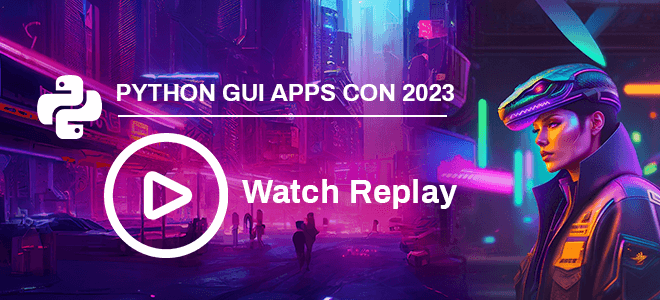# Build An Artificial Intelligence Solution With TensorFlow Library In A Delphi Windows AppAre you looking for an end-to-end open-source machine learning and deep learning platform, and build a nice GUI for them? You can deliver enterprise-grade AI solutions easily by combining TensorFlow and Python4Delphi library, inside Delphi and C++Builder.

TensorFlow is an open-source software library for high-performance numerical computation. Its flexible architecture allows easy deployment of computation across a variety of platforms (CPUs, GPUs, TPUs), and from desktops to clusters of servers to mobile and edge devices.

Originally developed by researchers and engineers from the Google Brain team within Google’s AI organization, it comes with strong support for machine learning and deep learning and the flexible numerical computation core is used across many other scientific domains.

## 1. Why TensorFlow?

Easy model building: Build and train ML models easily using intuitive high-level APIs like Keras with eager execution, which makes for immediate model iteration and easy debugging.

Robust ML production anywhere: Easily train and deploy models in the cloud, on-prem, in the browser, or on-device no matter what language you use.

Powerful experimentation for research: A simple and flexible architecture to take new ideas from concept to code, to state-of-the-art models, and publication faster.

## 2. Hands-On

This post will guide you on how to run the TensorFlow library to train neural networks and use Python for Delphi to display it in the Delphi Windows GUI app.

First, open and run our Python GUI using project `Demo1` from Python4Delphi with RAD Studio. Then insert the script into the lower `Memo`, click the `Execute script` button, and get the result in the upper Memo. You can find the `Demo1` source on GitHub. The behind the scene details of how Delphi manages to run your Python code in this amazing Python GUI can be found at this link.

This post will introduce you to how to perform pattern recognition on handwritten digits from scratch, and we will run it in Python GUI. In this tutorial, we will use the famous `MNIST` database of handwritten digits dataset, and we will train neural networks to classify the handwritten digits.

These are the steps to train your first neural networks in Python GUI by Python4Delphi:

• `import` library:
• Build the `tf.keras.Sequential` model by stacking layers. Choose an optimizer and loss function for training:
• For each example, the model returns a vector of “logits” or “log-odds” scores, one for each class.
• The `tf.nn.softmax` function converts these logits to “probabilities” for each class:
• The `losses.SparseCategoricalCrossentropy` loss takes a vector of `logits` and a `True` index and returns a scalar loss for each example.
• This loss is equal to the negative log probability of the true class: It is zero if the model is sure of the correct class. This untrained model gives probabilities close to random (1/10 for each class), so the initial loss should be close to `-tf.math.log(1/10) ~= 2.3`.
• The `model.fit` method adjusts the model parameters to minimize the loss:
• The `model.evaluate` method checks the models performance, usually on a “Validation-set” or “Test-set”.
• The image classifier is now trained to ~98% accuracy on this dataset. To learn more, read the TensorFlow tutorials. If you want your model to return a probability, you can wrap the trained model, and attach the `Softmax` layer to it:
• Run all the complete steps inside Python GUI:

Let’s compare the performance when it performs inside PyScripter IDE vs Python GUI by P4D:

Estimated time of arrival (ETA) for training neural networks in PyScripter IDE: `16m:37s`
vs
Estimated time of arrival (ETA) for training neural networks in Python GUI by P4D: `12m:25s`!

Congratulations, now you have learned how to run the TensorFlow library to train neural networks and use Python for Delphi to display it in the Delphi Windows GUI app! Now you can try lots more sophisticated examples from these documentations using the TensorFlow library and Python4Delphi.

Check out the `tensorflow` library for Python and use it in your projects: https://pypi.org/project/tensorflow/ and

Check out `Python4Delphi` which easily allows you to build Python GUIs for Windows using Delphi: https://github.com/pyscripter/python4delphi

 TensorFlow. (2023). Create production-grade machine learning models with TensorFlow. TensorFlow Website. tensorflow.org

 TensorFlow. (2023). TensorFlow 2 quickstart for beginners. TensorFlow Tutorials. tensorflow.org/tutorials/quickstart/beginner

 TensorFlow. (2023). TensorFlow 2 quickstart for experts. TensorFlow Tutorials. tensorflow.org/tutorials/quickstart/advanced

### Watch the Python GUI Apps Con 2023 sessions today!